# Semi-regular polyhedra

uniform polyhedra, Archimedean solids

A uniform polyhedron is a polyhedron all faces of which are regular polygons, while any vertex is related to all the other vertices by symmetry operations. Thus, the convex uniform polyhedra consist of the five Platonic solids along with those given in the Table, where \$ V \$ is the number of vertices, \$ E \$ the number of edges, \$ F \$ the number of faces, \$ F _ {k} \$ the number of \$ n _ {k} \$- gonal faces, \$ s \$ the number of faces meeting at each vertex, namely \$ s _ {1} \$ \$ n _ {1} \$- gonal faces, \$ s _ {2} \$ \$ n _ {2} \$- gonal faces, etc.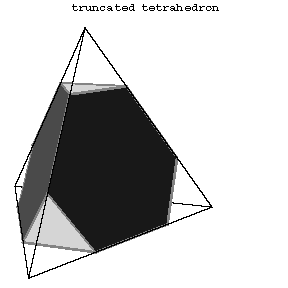Figure: s084300a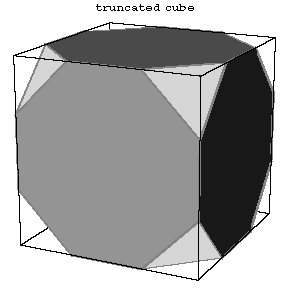Figure: s084300b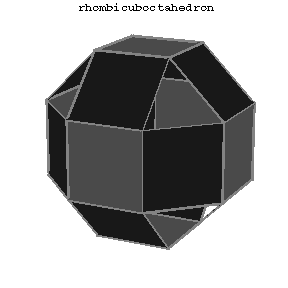Figure: s084300cFigure: s084300d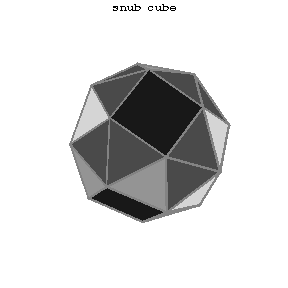Figure: s084300e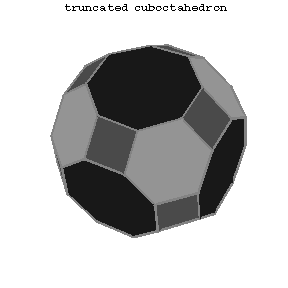Figure: s084300fFigure: s084300g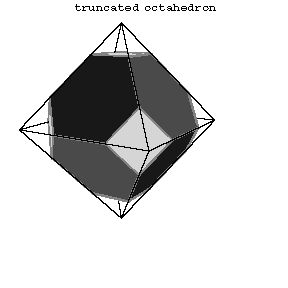Figure: s084300h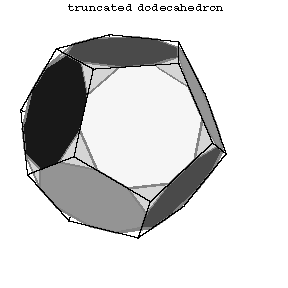Figure: s084300iFigure: s084300j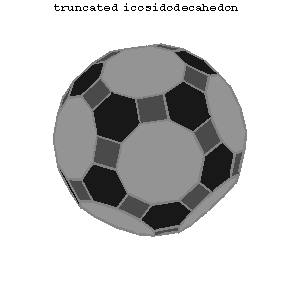Figure: s084300kFigure: s084300lFigure: s084300m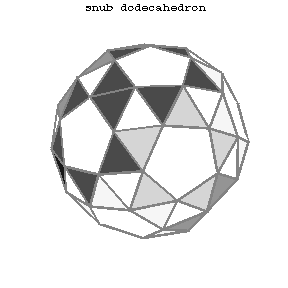Figure: s084300n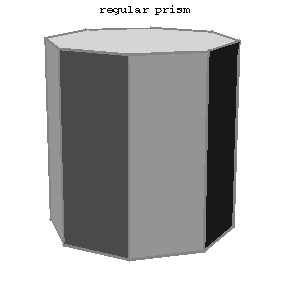Figure: s084300o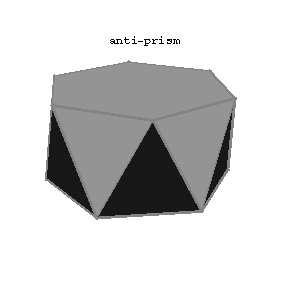Figure: s084300p

<tbody> </tbody>
 \$ 0 \$ \$ V \$ \$ E \$ \$ F \$ \$ n _ {1} \$ \$ n _ {2} \$ \$ n _ {3} \$ \$ F _ {1} \$ \$ F _ {2} \$ \$ F _ {3} \$ \$ s _ {1} \$ \$ s _ {2} \$ \$ s _ {3} \$ \$ s \$ Truncated tetrahedron 1 12 18 8 6 3 - 4 4 - 2 1 - 3 Truncated cube 2 24 36 14 8 3 - 6 8 - 2 1 - 3 Rhombicuboctahedron 3, 4 24 48 26 4 3 - 18 8 - 3 1 - 4 Snub cube 5 24 60 38 3 4 - 32 6 - 4 1 - 5 Truncated cuboctahedron 6 48 72 26 4 6 8 12 8 6 1 1 1 3 Cuboctahedron 7 12 24 14 3 4 - 8 6 - 2 2 - 4 Truncated octahedron 8 24 36 14 6 4 - 8 6 - 2 1 - 3 Truncated dodecahedron 9 60 90 32 10 3 - 12 20 - 2 1 - 3 Rhombicosidodecahedron 10 60 120 62 4 3 5 30 20 12 2 1 1 4 Truncated icosidodecahedron 11 120 180 62 4 6 10 30 20 12 1 1 1 3 Icosidodecahedron 12 30 60 32 3 5 - 20 12 - 2 2 - 4 Truncated icosahedron 13 60 90 32 6 5 - 20 12 - 2 1 - 3 Snub dodecahedron 14 60 150 92 3 5 - 80 12 - 4 1 - 5 Regular prism ( \$ n = 3, 5, 6,\dots \$) 15 \$ 2n \$ \$ 3n \$ \$ n+ 2 \$ 4 \$ n \$ - \$ n \$ 2 - 2 1 - 3 Antiprism ( \$ n = 4, 5, 6,\dots \$) 16 \$ 2n \$ \$ 4n \$ \$ 2n+ 2 \$ 3 \$ n \$ - \$ 2n \$ 2 - 3 1 - 4

The first 13 polyhedra in the Table are attributed to Archimedes (Fig. a, Fig. b, Fig. c, 5–14).

In addition to the 13 solids of Archimedes and the convex prisms (Fig.15) and antiprisms (Fig.16), there are non-convex prisms and antiprisms whose bases are "star polygons" , and 53 other non-convex uniform polyhedra.

How to Cite This Entry:
Semi-regular polyhedra. Encyclopedia of Mathematics. URL: http://encyclopediaofmath.org/index.php?title=Semi-regular_polyhedra&oldid=48666
This article was adapted from an original article by A.B. Ivanov (originator), which appeared in Encyclopedia of Mathematics - ISBN 1402006098. See original article